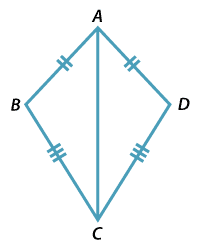### Congruence and kites$$ABCD$$ is a kite with $$AB = AD \ \text{and} \ CB = CD$$.

• $$\triangle ABC \equiv \triangle ADC$$.
• The diagonal AC is an axis of reflection symmetry of the kite.
• The axis AC bisects the vertex angles at A and C.
• $$\angle B = \angle D$$.